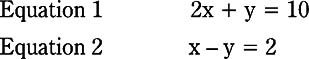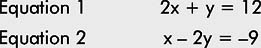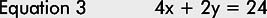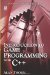# 2.7 Simultaneous Linear Equations

## 2.7 Simultaneous Linear Equations

The final subject to consider in this chapter is simultaneous linear equations, which is something of a mouthful when said aloud. The equations demonstrated so far contained only one unknown value that needed to be found, such asx+5=10.However, simultaneous equations are about solving for more than one unknown. Consider the following two equations:

• 2x + y = 10

• x y = 2

From these equations we must solve for both x and y. This means we must find the values of both x and y. There are two methods for reaching the answer: substitution and elimination.

### 2.7.1 Solving by Substitution

One of the most common methods for reaching the solution is substitution. This method requires us to solve one of the equations. In other words, we take one of the two equations and isolate a single term on one side of the equals sign, either x or y, and thereby express its relationship to every other term in that equation. This solution is then substituted into the second equation to solve for the other variable. Consider the following:First, consider equation 2:

• x y = 2

• x = 2 + y (x is isolated, and now we know x in relation to y)

Now substitute for x in equation 1.

• 2x + y = 10

• 2(2 + y) + y = 10 (x is substituted here by 2 + y)

• 4 + 3y = 10

• 3y = 10 4

• 3y = 6

• y = 2

Since x = 2 + y, and since y = 2, then x = 4.

Check this in equation 1:

• 8 + 2 = 10 (Correct)

### 2.7.2 Solving by Elimination

The second method for solving simultaneous equations is elimination, which is sometimes called the addition method. Here, both equations are combined into a single equation by either adding them together or subtracting them. The intention is to, by addition or subtraction, eliminate one of the other variable terms, either x or y, so that the resulting equation has only one term that must be solved. Afterward, the other term can be found through substitution. Consider the following equations:Adding the two equations together will result in y being eliminated since yy is 0. Remember, adding a negative number is the same as subtraction. The result follows.

• (2x + y = 10) + (x y = 2)

• 3x = 12

• x = 4

The value x = 4 can be substituted in either of the original equations, as follows:

• 2x + y = 10

• 8 + y = 10

• y = 10 8

• y = 2

So x = 4, and y = 2.

 Note There may be times when, given two equations with two unknowns, it may not be immediately possible to solve using the elimination method. This is because each equation may be such that, if added or subtracted, neither term (x or y) will be eliminated completely. In such cases, it is acceptable to multiply one or both equations until terms can be eliminated. For example:Here, if these equations are added the result will still have x and y. Neither term will have been eliminated because 2x + x = 3x and −2y + y = −y. However, equation 1 can be multiplied by 2 to produce a third equation, as follows.Now one term can be cancelled with addition: (4x + 2y = 24) + (x− 2y = − 9) 5x = 15 x = 3 Now substitute in equation 1: 2x + y = 12 6 + y = 12 y = 12 − 6 y = 6 So: x = 3 and y = 6 Check this in equation 2: x − 2y = −9 3 − 12 = −9 (Correct)Introduction to Game Programming with C++ (Wordware Game Developers Library)
ISBN: 1598220322
EAN: 2147483647
Year: 2007
Pages: 225
Authors: Alan Thorn

Similar book on Amazon

flylib.com © 2008-2017.
If you may any questions please contact us: flylib@qtcs.net